# Precalculus : Solving Right Triangles

## Example Questions

2 Next →

### Example Question #11 : Trigonometric Applications

Find the area of the given isosceles triangle: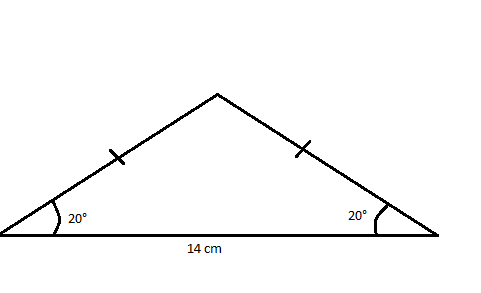Possible Answers: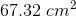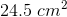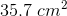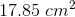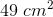Correct answer:Explanation:

The first step is to divide this isosceles triangle into 2 right triangles, making it easier to solve: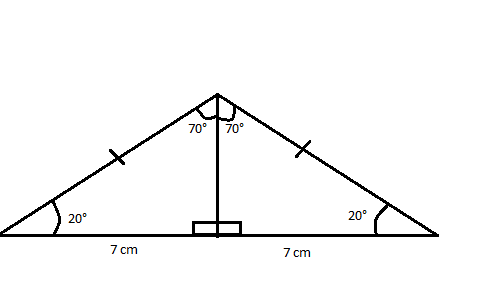The equation for area is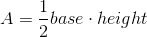We already know the base, so we need to solve for height to get the area.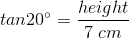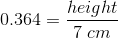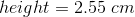Then we plug in all values for the equation: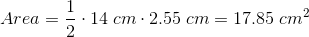### Example Question #12 : Trigonometric Applications

Find the area of the given isosceles triangle: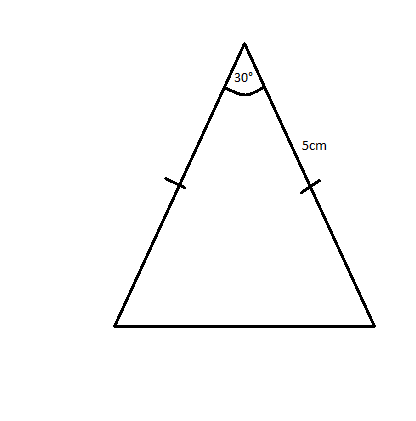Possible Answers: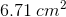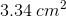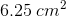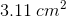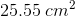Correct answer:Explanation:

The first step toward finding the area is to divide this isosceles triangle into two right triangles: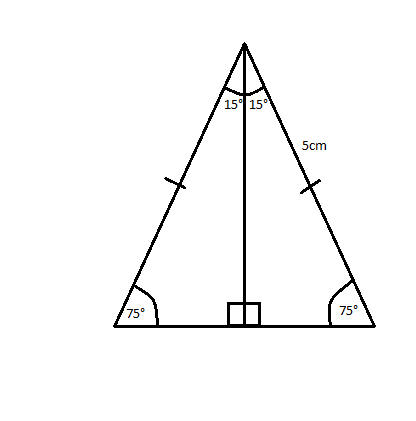Trigonometric ratios can be used to find both the height and the base, which are needed to calculate area: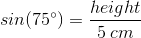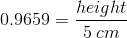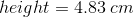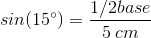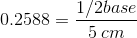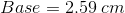With both of those values calculated, we can now calculate the area of the triangle: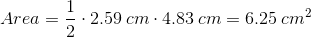### Example Question #351 : Pre Calculus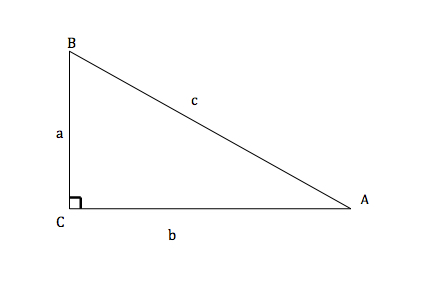Solve the right triangle given that a=5, b=12, and A=22.620°

Possible Answers:

None of these answers are correct.

B=90°

C=67.380°

c=169

B=90°

C=67.380°

c=13

B=67.380°

C=90°

c=13

B=67.380°

C=90°

c=17

Correct answer:

B=67.380°

C=90°

c=13

Explanation:C is given as 90°.

A is given as 22.620°

a is given as 5

b is given as 12Therefore...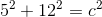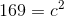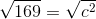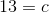All angles of a triangle add up to equal 180°.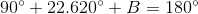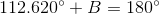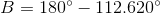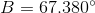2 Next →

### All Precalculus Resources